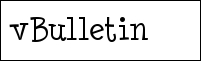用户名 记住信息 密码
 注册账号 论坛帮助 会员列表 日历事件 搜索 今日新帖 标记版面已读

 关键词: 搜索整个帖子 只搜索标题

 用户名: 查找该用户发表的所有帖子 查找该用户发表的所有主题 精确匹配用户名

 请输入图像中的六位数字或字母。查找包含前缀的主题 (任意前缀) (无前缀) [公告] [申请] [讨论] [MATLAB基础] [MATLAB数学相关] [MATLAB图像处理] [MATLAB GUI] [MATLAB信号处理] [MATLAB通信系统] [MATLAB混合编程] [MATLAB工具箱] [MATLAB毕业设计] [Simulink相关] [求助] [讨论] [文章] [资料] [分享] [招聘] [其它] 查找主题 至少 最多 回复 查找帖子 任何日期 您上次访问 昨天 1 周前 2 周前 1 月前 3 月前 6 月前 1 年前 早于 晚于 结果排序 相关性 标题 回复人数 查看人数 主题发表日期 最近发表日期 用户名 版面 降序 升序 结果显示 主题 帖子 按标签搜索 标签: 搜索论坛 搜索所有开放的版面 搜索收藏的版面 论坛管理     论坛公告     论坛事务 特色讨论区:工程数学软件-不仅仅是MATLAB!     MATLAB论坛         MATLAB算法讨论         MATLAB RSS新闻聚合测试区         MATLAB技术文章     MathCAD论坛     Mathematica论坛     Maple论坛     Origin论坛     ANSYS论坛     LabVIEW论坛 人工智能|模式识别|机器学习|数字图像处理|SAR图像处理-不仅仅是MATLAB!     遗传算法(GA)     人工神经网络(ANN)     模拟退火算法(SAA)     人工免疫系统(AIS)     数字图像处理|SAR图像处理 编程论坛—学习编程的艺术--不仅仅是MATLAB!     C语言     C++     JAVA     硬件编程 基础科学-不仅仅是MATLAB!     数学         数学建模专区     物理学     化学 科研交流区-不仅仅是MATLAB!     英语学习     学术杂谈 其他-不仅仅是MATLAB!     广告与招聘     论坛水库 包含子版面

 -- 简体中文 -- 繁體中文 -- English (US) 联系我们 - MATLAB中国论坛|MATLAB爱好者之家 - 论坛存档 - 返回顶端